# Hume AI is a research lab and technology company. Our mission is to ensure that artificial intelligence is built to serve human goals and emotional well-being.self.__wrap_b=(t,r,e)=>{e=e||document.querySelector(`[data-br="\${t}"]`);let s=e.parentElement,a=T=>e.style.maxWidth=T+"px";e.style.maxWidth="";let n=s.clientWidth,u=s.clientHeight,o=n/2-.25,c=n+.5,p;if(n){for(a(o),o=Math.max(e.scrollWidth,o);o+1<c;)p=Math.round((o+c)/2),a(p),s.clientHeight===u?c=p:o=p;a(c*r+n*(1-r))}e.__wrap_o||(typeof ResizeObserver!="undefined"?(e.__wrap_o=new ResizeObserver(()=>{self.__wrap_b(0,+e.dataset.brr,e)})).observe(s): false&&false)};self.__wrap_b("undefined",1)

## Visionself.__wrap_b=(t,r,e)=>{e=e||document.querySelector(`[data-br="\${t}"]`);let s=e.parentElement,a=T=>e.style.maxWidth=T+"px";e.style.maxWidth="";let n=s.clientWidth,u=s.clientHeight,o=n/2-.25,c=n+.5,p;if(n){for(a(o),o=Math.max(e.scrollWidth,o);o+1<c;)p=Math.round((o+c)/2),a(p),s.clientHeight===u?c=p:o=p;a(c*r+n*(1-r))}e.__wrap_o||(typeof ResizeObserver!="undefined"?(e.__wrap_o=new ResizeObserver(()=>{self.__wrap_b(0,+e.dataset.brr,e)})).observe(s): false&&false)};self.__wrap_b("undefined",1)

Our vision is a world where AI translates scientific insights into new ways to improve human emotional experience. Emotional awareness is the missing ingredient needed to build social media algorithms that optimize for user well-being instead of engagement metrics, digital assistants that respond with more nuance to your present state of mind, technologies that help animators create relatable characters, and much more.

## Valuesself.__wrap_b=(t,r,e)=>{e=e||document.querySelector(`[data-br="\${t}"]`);let s=e.parentElement,a=T=>e.style.maxWidth=T+"px";e.style.maxWidth="";let n=s.clientWidth,u=s.clientHeight,o=n/2-.25,c=n+.5,p;if(n){for(a(o),o=Math.max(e.scrollWidth,o);o+1<c;)p=Math.round((o+c)/2),a(p),s.clientHeight===u?c=p:o=p;a(c*r+n*(1-r))}e.__wrap_o||(typeof ResizeObserver!="undefined"?(e.__wrap_o=new ResizeObserver(()=>{self.__wrap_b(0,+e.dataset.brr,e)})).observe(s): false&&false)};self.__wrap_b("undefined",1)

We’re committed to paving the way for prosocial AI while staying true to our values and principles:

### Empathyself.__wrap_b=(t,r,e)=>{e=e||document.querySelector(`[data-br="\${t}"]`);let s=e.parentElement,a=T=>e.style.maxWidth=T+"px";e.style.maxWidth="";let n=s.clientWidth,u=s.clientHeight,o=n/2-.25,c=n+.5,p;if(n){for(a(o),o=Math.max(e.scrollWidth,o);o+1<c;)p=Math.round((o+c)/2),a(p),s.clientHeight===u?c=p:o=p;a(c*r+n*(1-r))}e.__wrap_o||(typeof ResizeObserver!="undefined"?(e.__wrap_o=new ResizeObserver(()=>{self.__wrap_b(0,+e.dataset.brr,e)})).observe(s): false&&false)};self.__wrap_b("undefined",1)

AI privy to cues of our emotions should serve our emotional well-being.

### Beneficenceself.__wrap_b=(t,r,e)=>{e=e||document.querySelector(`[data-br="\${t}"]`);let s=e.parentElement,a=T=>e.style.maxWidth=T+"px";e.style.maxWidth="";let n=s.clientWidth,u=s.clientHeight,o=n/2-.25,c=n+.5,p;if(n){for(a(o),o=Math.max(e.scrollWidth,o);o+1<c;)p=Math.round((o+c)/2),a(p),s.clientHeight===u?c=p:o=p;a(c*r+n*(1-r))}e.__wrap_o||(typeof ResizeObserver!="undefined"?(e.__wrap_o=new ResizeObserver(()=>{self.__wrap_b(0,+e.dataset.brr,e)})).observe(s): false&&false)};self.__wrap_b("undefined",1)

AI should be deployed only if its benefits substantially outweigh its costs.

### Scientific Legitimacyself.__wrap_b=(t,r,e)=>{e=e||document.querySelector(`[data-br="\${t}"]`);let s=e.parentElement,a=T=>e.style.maxWidth=T+"px";e.style.maxWidth="";let n=s.clientWidth,u=s.clientHeight,o=n/2-.25,c=n+.5,p;if(n){for(a(o),o=Math.max(e.scrollWidth,o);o+1<c;)p=Math.round((o+c)/2),a(p),s.clientHeight===u?c=p:o=p;a(c*r+n*(1-r))}e.__wrap_o||(typeof ResizeObserver!="undefined"?(e.__wrap_o=new ResizeObserver(()=>{self.__wrap_b(0,+e.dataset.brr,e)})).observe(s): false&&false)};self.__wrap_b("undefined",1)

Applications of AI should be supported by collaborative, rigorous, and inclusive science.

### Emotional Primacyself.__wrap_b=(t,r,e)=>{e=e||document.querySelector(`[data-br="\${t}"]`);let s=e.parentElement,a=T=>e.style.maxWidth=T+"px";e.style.maxWidth="";let n=s.clientWidth,u=s.clientHeight,o=n/2-.25,c=n+.5,p;if(n){for(a(o),o=Math.max(e.scrollWidth,o);o+1<c;)p=Math.round((o+c)/2),a(p),s.clientHeight===u?c=p:o=p;a(c*r+n*(1-r))}e.__wrap_o||(typeof ResizeObserver!="undefined"?(e.__wrap_o=new ResizeObserver(()=>{self.__wrap_b(0,+e.dataset.brr,e)})).observe(s): false&&false)};self.__wrap_b("undefined",1)

AI should be prevented from treating human emotion as a means to an end.

### Inclusivityself.__wrap_b=(t,r,e)=>{e=e||document.querySelector(`[data-br="\${t}"]`);let s=e.parentElement,a=T=>e.style.maxWidth=T+"px";e.style.maxWidth="";let n=s.clientWidth,u=s.clientHeight,o=n/2-.25,c=n+.5,p;if(n){for(a(o),o=Math.max(e.scrollWidth,o);o+1<c;)p=Math.round((o+c)/2),a(p),s.clientHeight===u?c=p:o=p;a(c*r+n*(1-r))}e.__wrap_o||(typeof ResizeObserver!="undefined"?(e.__wrap_o=new ResizeObserver(()=>{self.__wrap_b(0,+e.dataset.brr,e)})).observe(s): false&&false)};self.__wrap_b("undefined",1)

The benefits of AI should be shared by people from diverse backgrounds.

### Transparencyself.__wrap_b=(t,r,e)=>{e=e||document.querySelector(`[data-br="\${t}"]`);let s=e.parentElement,a=T=>e.style.maxWidth=T+"px";e.style.maxWidth="";let n=s.clientWidth,u=s.clientHeight,o=n/2-.25,c=n+.5,p;if(n){for(a(o),o=Math.max(e.scrollWidth,o);o+1<c;)p=Math.round((o+c)/2),a(p),s.clientHeight===u?c=p:o=p;a(c*r+n*(1-r))}e.__wrap_o||(typeof ResizeObserver!="undefined"?(e.__wrap_o=new ResizeObserver(()=>{self.__wrap_b(0,+e.dataset.brr,e)})).observe(s): false&&false)};self.__wrap_b("undefined",1)

People affected by AI should have enough data to make decisions about its use.

### Consentself.__wrap_b=(t,r,e)=>{e=e||document.querySelector(`[data-br="\${t}"]`);let s=e.parentElement,a=T=>e.style.maxWidth=T+"px";e.style.maxWidth="";let n=s.clientWidth,u=s.clientHeight,o=n/2-.25,c=n+.5,p;if(n){for(a(o),o=Math.max(e.scrollWidth,o);o+1<c;)p=Math.round((o+c)/2),a(p),s.clientHeight===u?c=p:o=p;a(c*r+n*(1-r))}e.__wrap_o||(typeof ResizeObserver!="undefined"?(e.__wrap_o=new ResizeObserver(()=>{self.__wrap_b(0,+e.dataset.brr,e)})).observe(s): false&&false)};self.__wrap_b("undefined",1)

AI should be deployed only with the informed consent of the people whom it affects.

All of our work is guided by the Ethical Guidelines of The Hume Initiative

## Teamself.__wrap_b=(t,r,e)=>{e=e||document.querySelector(`[data-br="\${t}"]`);let s=e.parentElement,a=T=>e.style.maxWidth=T+"px";e.style.maxWidth="";let n=s.clientWidth,u=s.clientHeight,o=n/2-.25,c=n+.5,p;if(n){for(a(o),o=Math.max(e.scrollWidth,o);o+1<c;)p=Math.round((o+c)/2),a(p),s.clientHeight===u?c=p:o=p;a(c*r+n*(1-r))}e.__wrap_o||(typeof ResizeObserver!="undefined"?(e.__wrap_o=new ResizeObserver(()=>{self.__wrap_b(0,+e.dataset.brr,e)})).observe(s): false&&false)};self.__wrap_b("undefined",1)

We are a team of scientists, engineers, and business leaders driven by the pursuit of understanding human emotion and improving human well-being.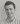Alan Cowen, PhD

CEO + Chief Scientist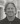Dacher Keltner, PhD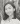Janet Ho

Chief Operating Officer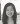Lauren Kim

Senior Machine Learning Engineer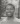Michael Opara

Senior Machine Learning Engineer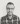Panagiotis Tzirakis, PhD

Senior AI Research Scientist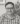Jacob Metrick

Principal Software Engineer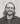Jeffrey Brooks, PhD

Computational Psychologist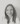Alice Baird, PhD

AI Research Scientist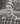Chris Gregory

Senior Machine Learning Engineer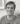Garrett Boseck

Senior Software Engineer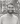Product Manager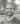Chris Gagne, PhD

AI Research Scientist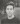Zach Krall

Creative Technologist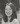Yini Shi

Senior Frontend Software Engineer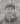Haoqi Li, PhD

AI Research Scientist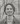Nathan Leung

Machine Learning Engineer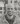Franc Camps-Febrer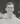Jake Marlo

Product Operations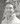Moses Oh

Machine Learning Engineer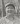James Cha-Earley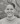Zachary Greathouse

Senior Developer Relations Engineer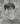Michael Wu

Machine Learning Engineer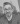Matt Forte

Creative Producer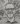Brenton McMenamin

Senior Data Engineer